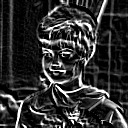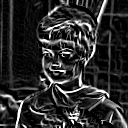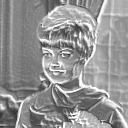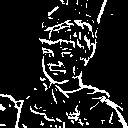##Fred's ImageMagick Scripts

 Licensing: Copyright © Fred Weinhaus My scripts are available free of charge for non-commercial (non-profit) use, ONLY. For use of my scripts in commercial (for-profit) environments or non-free applications, please contact me (Fred Weinhaus) for licensing arrangements. My email address is fmw at alink dot net. If you: 1) redistribute, 2) incorporate any of these scripts into other free applications or 3) reprogram them in another scripting language, then you must contact me for permission, especially if the result might be used in a commercial or for-profit environment. Usage, whether stated or not in the script, is restricted to the above licensing arrangements. It is also subject, in a subordinate manner, to the ImageMagick license, which can be found at: http://www.imagemagick.org/script/license.php Please read the Pointers For Use on my home page to properly install and customize my scripts.

## GRADIENT

 Applies a gradient filter to an image.

Download Script

last modified: December 15, 2018

 USAGE: gradient [-k kind] [-f filter] [-v version] [t thresh] [-m mix] [-b bias] infile outfile USAGE: gradient [-h or -help] -k ...... kind ..... 1=approximate and 2=exact; default=1 -f ...... filter ... 1=Prewitt (equal wts); 2=Sobel (binomial wts); .................... default=1 -v ...... version .. 1=one-sided gradient and 2=two-sided gradient; .................... default=1 -t ...... thresh ... threshold percent for binarization; .................... thresh=integer 0 to 100; default="" (none) -m ...... mix ...... mixing percent with original image; .................... mix=integer 0 to 100; default=100 -b ...... bias ..... bias to add to result to brighten only; .................... bias=integer 0 to 100; default=0 -h ................. get help information -help .............. get help information PURPOSE: To apply a gradient filter to an image. DESCRIPTION: GRADIENT applies a 3x3 gradient magnitude filter to an image to extract the edges in the image. It is a type of high pass filter. Two kinds of gradients can be applied, approximate or exact. Two different filter forms can be used, Prewitt's, which uses equal weights or Sobel's, which uses binomial coefficient weights. The gradient can be thresholded into a binary output. The gradient can be blended (mixed) with the input image, which will cause some darkening. Therefore a bias can be added to compensate and brighten the output. Blending is done according to F = (1-m)*I + m*G, where I is the original image, G is the gradient filtered image and m = mix/100. In this case, blending does not cause image sharpening and is therefore not very useful. ARGUMENTS: -k kind is the kind of gradient, either approximate (quicker) or exact (slower). A value of kind=1 is approximate and a value o kind=2 is exact. The default is kind=1. The gradient magnitude is formed by computing both x and y first derivative convolutions on the image. For kind=1, the absolute value of each component result is computed and then added together to form the result. For kind=2, each component is squared, added together and finally the square root is computed to form the result. There is only marginal differences between the approximate and exact gradients. -f filter is the form of the filter. Two different filter forms can be used, Prewitt's, which uses equal weights or Sobel's, which uses binomial coefficient weights. A value of filter=1 is Prewitt's and a value of filter=2 is Sobel's. The default is filter=1. There is only marginal differences between the two filter form gradients. Prewitt's Derivatives: DX: -1 0 1 -1 0 1 -1 0 1 DY:  1  1  1  0  0  0 -1 -1 -1 Sobel's Derivatives: DX: -1 0 1 -2 0 2 -1 0 1 DY:  1  2  1  0  0  0 -1 -2 -1 -v version identifies whether to use a one-sided or two-sided gradient. The one-sided gradient (original development) is limited by IM's clipping when not in HDRI mode. The two-sided gradient (new addition) gives the full proper gradient by using a bias to avoid clipping. The default=1 for backward compatibility, but the version 2 is more correct and recommended. -t thresh is the thresholding percentage used to create a binary gradient edge image. Values range from 0 to 100. A higher value will result in fewer edges in the resulting image. -m mix is the percentage mixing factor used to blend the gradient with the original image. A value of mix=0, results in the original image. A value of mix=100 results in a pure gradient image. Mixing has an effect of darkening the image, because the gradient has only positive edges. Therefore, a bias is provided to compensate for this effect. Consequently, mixing is not very effective when extracting the gradient edges. It does not cause sharpening of the image. -b bias is a percentage to brighten the image to compensate for darkening due to mixing. Values for bias are integers ranging from 0 to 100 (percent). CAVEAT: No guarantee that this script will work on all platforms, nor that trapping of inconsistent parameters is complete and foolproof. Use At Your Own Risk.

### EXAMPLES

 Gradient Of A True Color Image Original Image Prewitt Approx.Gradient Prewitt ExactGradientOriginal Image Sobel Approx.Gradient Sobel ExactGradientGradient Of A Grayscale Image Original Image Prewitt Approx.Gradient Prewitt ExactGradientOriginal Image Sobel Approx.Gradient Sobel ExactGradientGradient Blending Original Image Prewitt Approx.Gradient Arguments: -k 1 -f 1 -m 50 -b 20Original Image Prewitt Approx.Gradient Arguments: -k 1 -f 1 -m 50 -b 20Gradient Thresholding Original Image Prewitt Approx.Gradient Arguments: -k 1 -f 1 -t 50Gradient Version Comparison Original Image Arguments: -k 1 -f 1 -v 1 Arguments: -k 1 -f 1 -v 2What the script does is as follows: Computes each of the x and y first derivatives using a convolution filter. Combines the derivatives to form the gradient. Optionally, thresholds the gradient or Optionally, mixes the gradient with the original This is equivalent to the following IM commands for the case of the approximate Prewitt gradient dx="-1,0,1,-1,0,1,-1,0,1" dy="1,1,1,0,0,0,-1,-1,-1" convert \$infile -convolve "\$dx" -fx "abs(u)" \$tmpX convert \$infile -convolve "\$dy" -fx "abs(u)" \$tmpY convert \$tmpX \$tmpY -compose plus -composite \$outfile